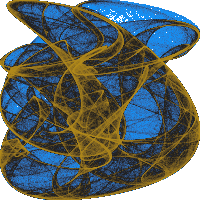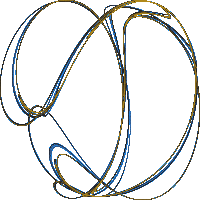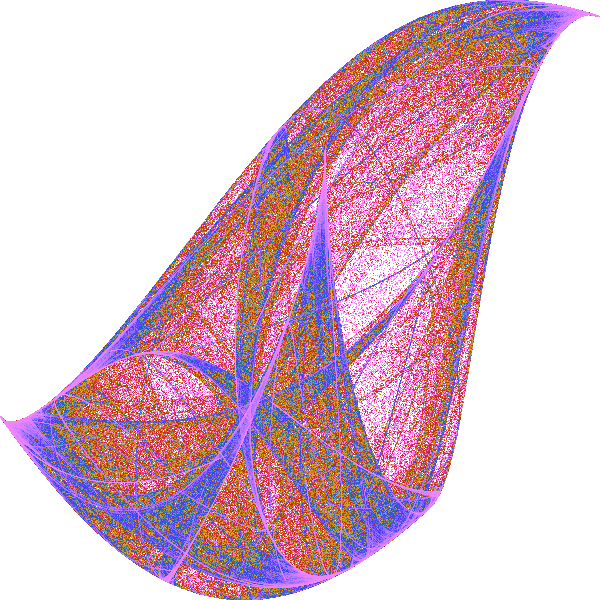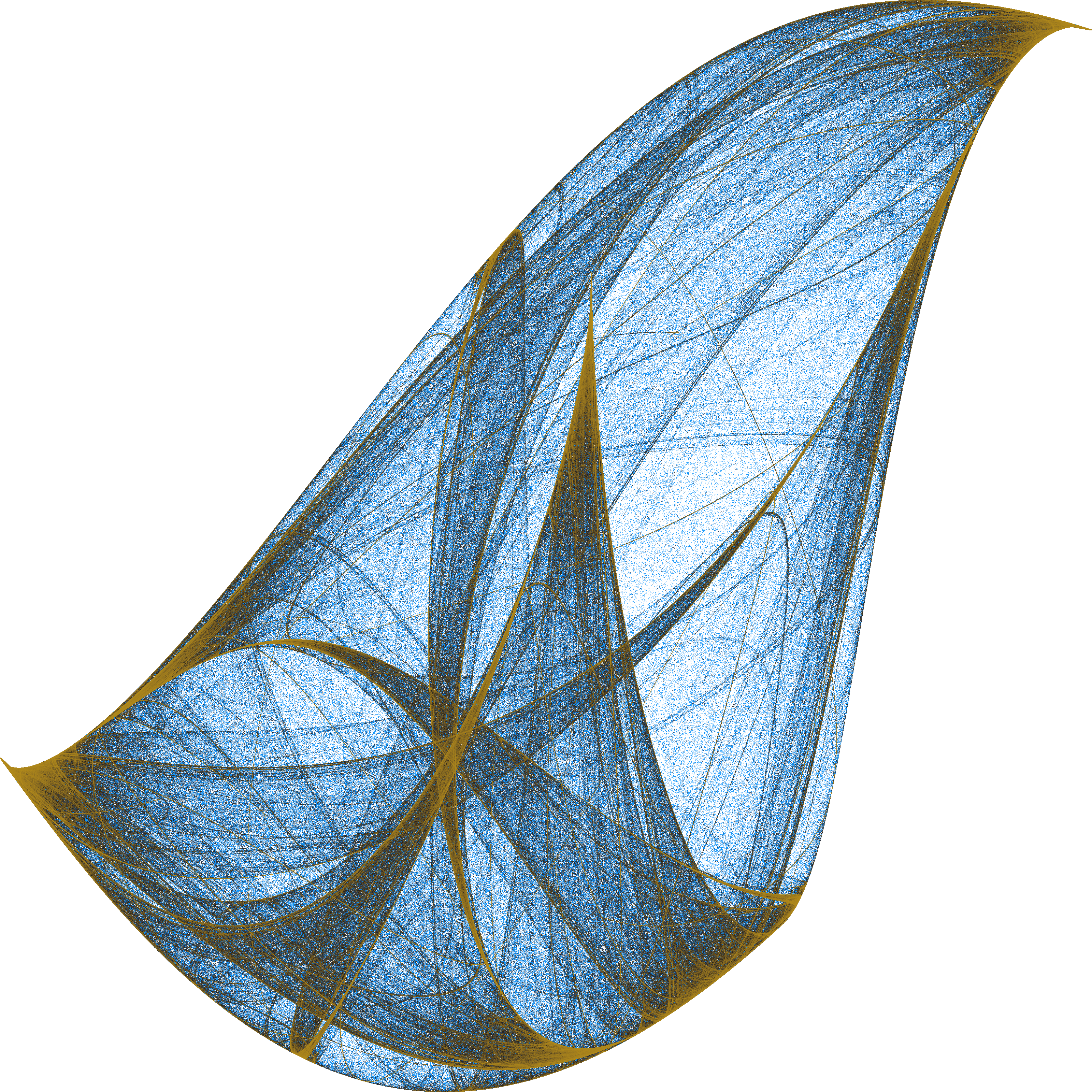# Creating images of strange attractors

My fascination with mathematics and generative art continues. Here I want to describe my current workflow for making images of strange attractors.

In mathematics, an attractor is a set of numerical values toward which a system tends to evolve, for a wide variety of starting conditions of the system. This means that system values that get close enough to the attractor values remain close even if slightly disturbed. For a strange attractor the movement around the attractor tend to form an interesting patterns.

For this example we will use the Clifford attractor, which is defined by the following set of equations:

\begin{aligned} x_{n+1} &= \sin(a\cdot y_n)+c\cdot\cos(a\cdot x_n)\\ y_{n+1} &= \sin(b\cdot x_n) + d\cdot\cos(b\cdot y_n) \end{aligned}

Given the point $$(x_n, y_n)$$ you can calculate the point $$(x_{n+1}, y_{n+1})$$. The values $$a$$, $$b$$, $$c$$, and $$d$$ are parameters. Calling the function on its own output many times will give you a trajectory. For some values of the parameters, this trajectory will trace out an interesting pattern. To make those patterns visible I use the Python module datashader to turn the collection of points into a color-coded image, where the color represents the number of points that fall within a given pixel. This can produce images that look like this:To discover new shapes, I set the values of the parameters to be random numbers between -2 and 2. Once that is done I set the initial value of $$(x,y)$$ to be $$(0,0)$$ and start iterating the system – that is I call the function on its own output again and again – and hope that a pattern emerges. Not all values for the parameters will create interesting patterns.

To make the process of discovery fast I generate images that are only 200 by 200 pixels, and iterate 2 million times. This gives me enough information to decide which pattern I like the most. Below I’ve given examples of pattern that emerges the last time I did this. The whole process took just a few minutes. Hovering over the small images will bring them in focus.Once I have my favorite pattern I need to decide on a color scheme. For this, I like to create images in a resolution that matches what I need for creating merchandise. Currently, that is 7.200 by 7.200 pixels with 200 million iterations of the function system. However, that would take a long time to load, so for this post, I’ve created them using just 600 by 600 pixels and 2 million iterations. The results are shown below:This gives you an idea that the shape looks like in different colors. However, the colors in the image above doesn’t quite do it justice, so I’ve included a 3.200 by 3.200 pixel image of the color I ended up using below:And thats it. I love that equation can produce a seemingly endless number of patterns. It genuinely feels like I’m discovering new shapes every time I run this code. And there are so many more (infinitely many?) iterated function systems to try out. I see myself playing around with this stuff for a long time to come.

To force myself to make the final design decision when creating these images, I’ve created a profile on Society6. This allows me to sell merchandise without worrying about storage and production. It will be interesting to see if anything comes of it. If nothing else it is motivating thing thinnk that the images can end up on real physical things. The pattern shown above is available for poster prints and other merchandise together with a collection of other patterns at my Society6 profile.